# Printable Number GridUpdated on Nov 24, 2022By Printablee Team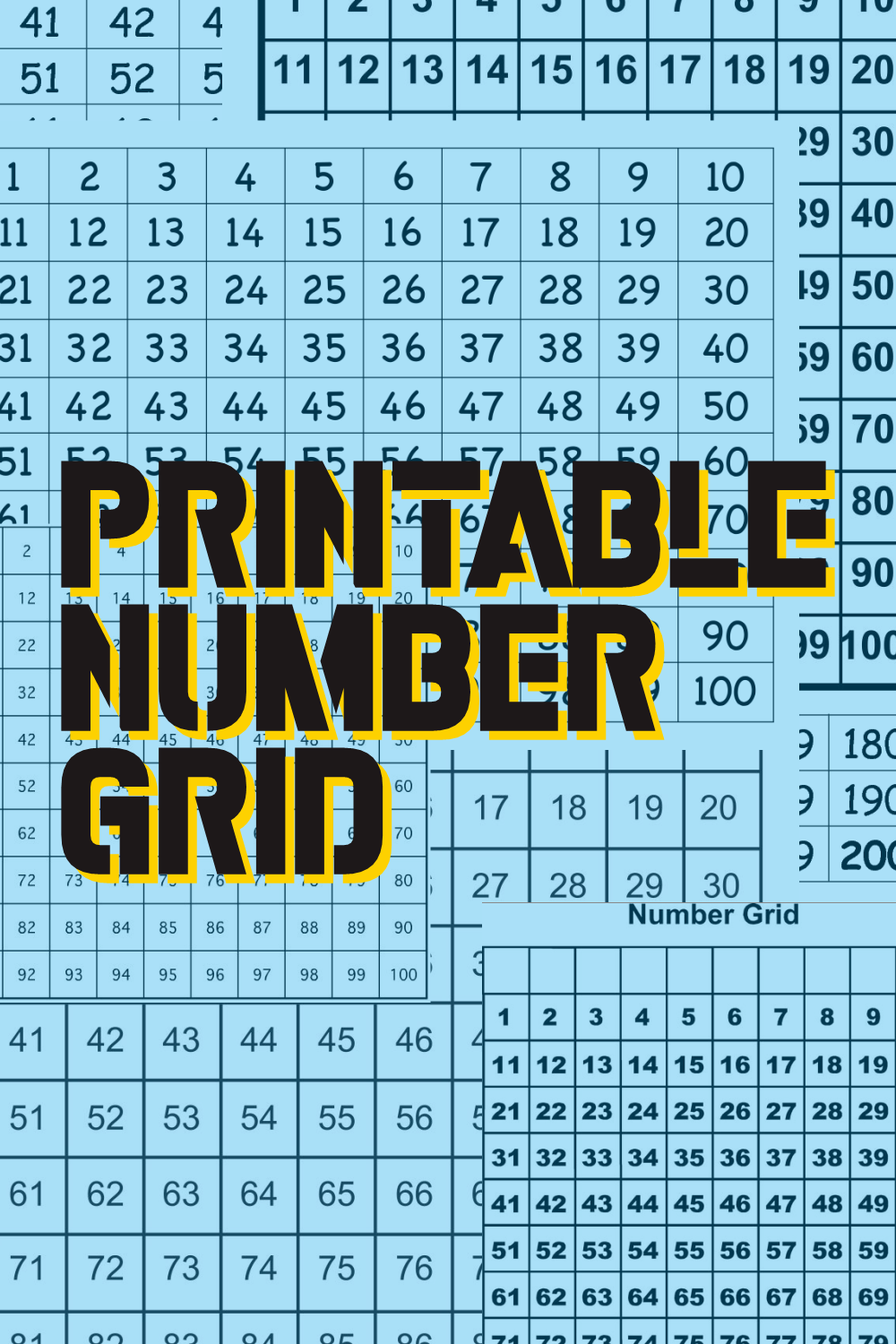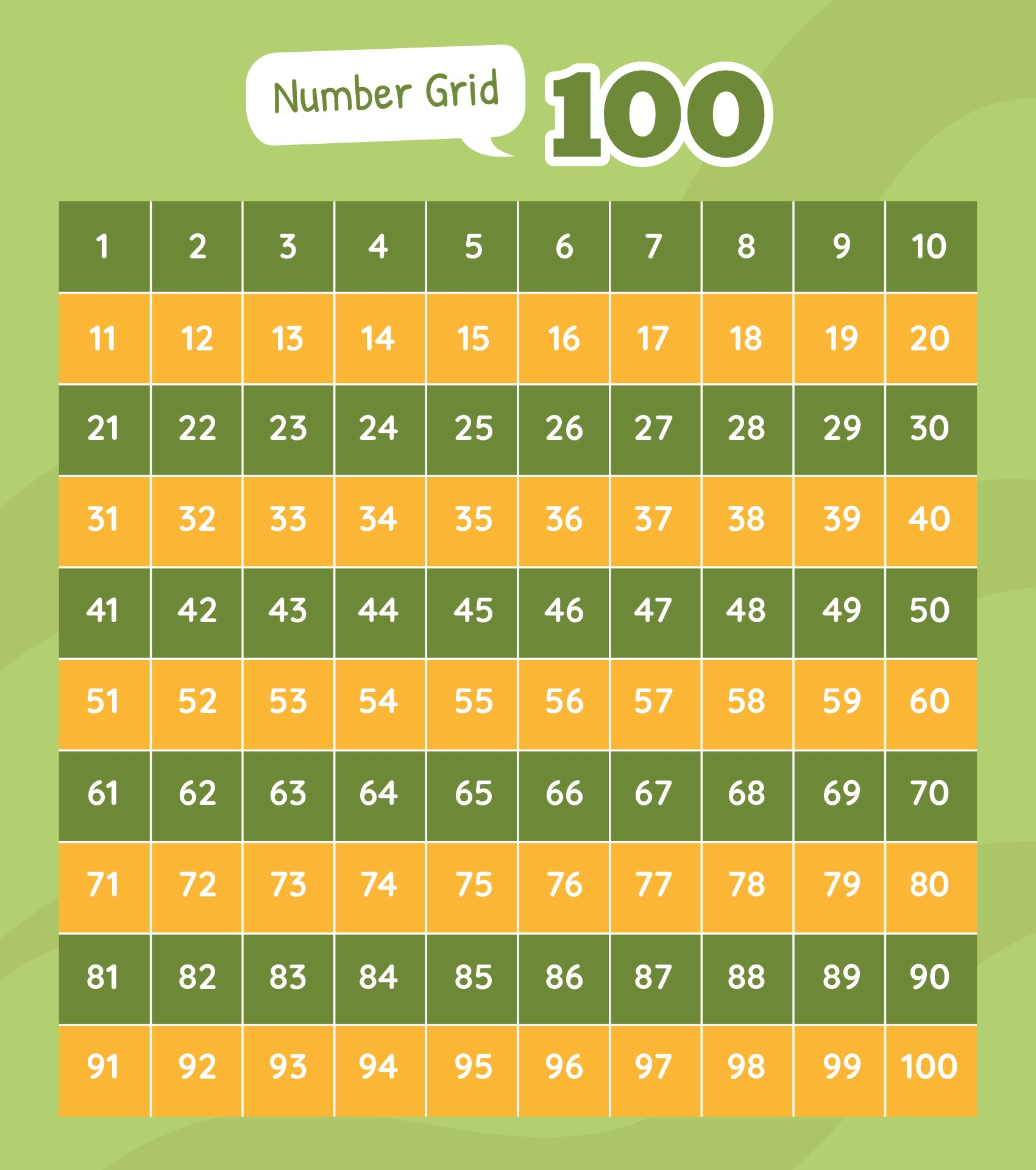## What is Number Grid?

Number grids, often known as hundred charts or number squares, are grids with rows and columns of numbers. The conventional number grid is normally a 10x10 grid with numbers ranging from 1 to 100. Number grids, on the other hand, may be adjusted to fulfill specific learning objectives, such as focusing on prime numbers or multiples of a given number.

Number grids are effective mathematical tools that can aid students in honing their analytical, problem-solving, and numerical reasoning abilities. These grids give numbers and patterns an organized visual representation, allowing pupils to study and comprehend a variety of mathematical ideas. Upper elementary kids can open up a world of mathematical possibilities by becoming experts at deciphering number grids.

## How to Understand the Number Grid Pattern?

Number grids are used to visualize counting patterns. Students can see the sequential order of numerals as they go from left to right and top to bottom. This helps pupils build number sense and reinforces the concept of counting by ones.

Skip counting, in which children count by a set increment, is likewise facilitated by number grids. Students can identify the numbers that follow a specific skip-counting sequence by analyzing the patterns in the grid. This ability is essential for mastering multiplication and division.

Prime and composite numbers can be identified using number grids. Students can color or highlight prime integers to better understand the distribution of primes on the grid. This practice encourages a more in-depth grasp of number characteristics and builds the groundwork for more complex mathematical ideas.

Number grids are a useful tool for investigating multiples and factors. By tracing the rows or columns, students may determine the numbers that are multiples of a certain factor. They may also find the factors of a number by noting the spots in the grid where that number appears. This improves pupils' understanding of multiplication and division connections.

Number grids demonstrate mathematical patterns. Examining diagonal, horizontal, or vertical number sequences, for example, can reveal additional patterns. Similarly, multiplication patterns may be detected by examining the distribution of numbers inside the grid. Understanding these patterns helps pupils improve their computational and mental math skills.

## How Does Number Grid Activity Help Develop Problem-solving Skills?

Number grids may be used to design puzzles and challenges that force pupils to use their problem-solving abilities. Students, for example, may be challenged with locating a certain number pattern or solving a missing number problem inside the grid. These exercises foster critical thinking, logical reasoning, and persistence.

Number grids can help pupils enhance their algebraic reasoning. Students can find numerical relationships and investigate equations by noticing patterns in the grid. They can study and represent algebraically how numbers vary over rows, columns, and diagonals. This allows for a more in-depth comprehension of variables, expressions, and equations.

Geometry and number grids may coexist. By choosing a series of integers, students may utilize the grid to make geometric forms like rectangles, squares, or triangles. Geometric visualization and spatial thinking are improved as a result of the integration of geometry and number grids.

You may create data sets from number grids to use in statistical analysis. By choosing particular numbers from the grid, students may gather data and use that data to make graphs or charts. Students gain knowledge and abilities in data gathering, representation, and interpretation through this practical approach to data analysis.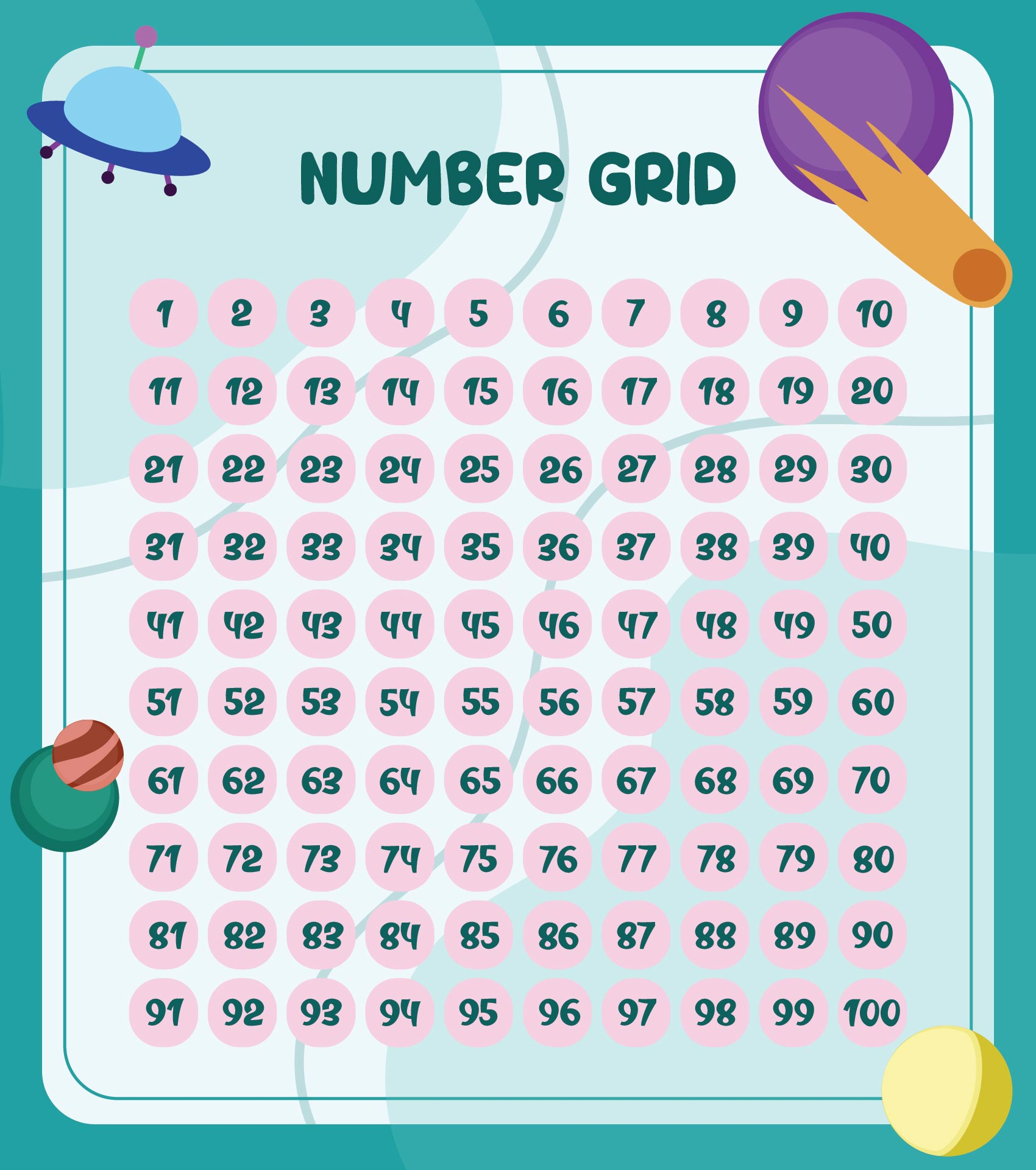We also have more printable number you may like:
Printable 120 Number Grid
Printable Block Numbers 1 10
Winter Printable Color By Number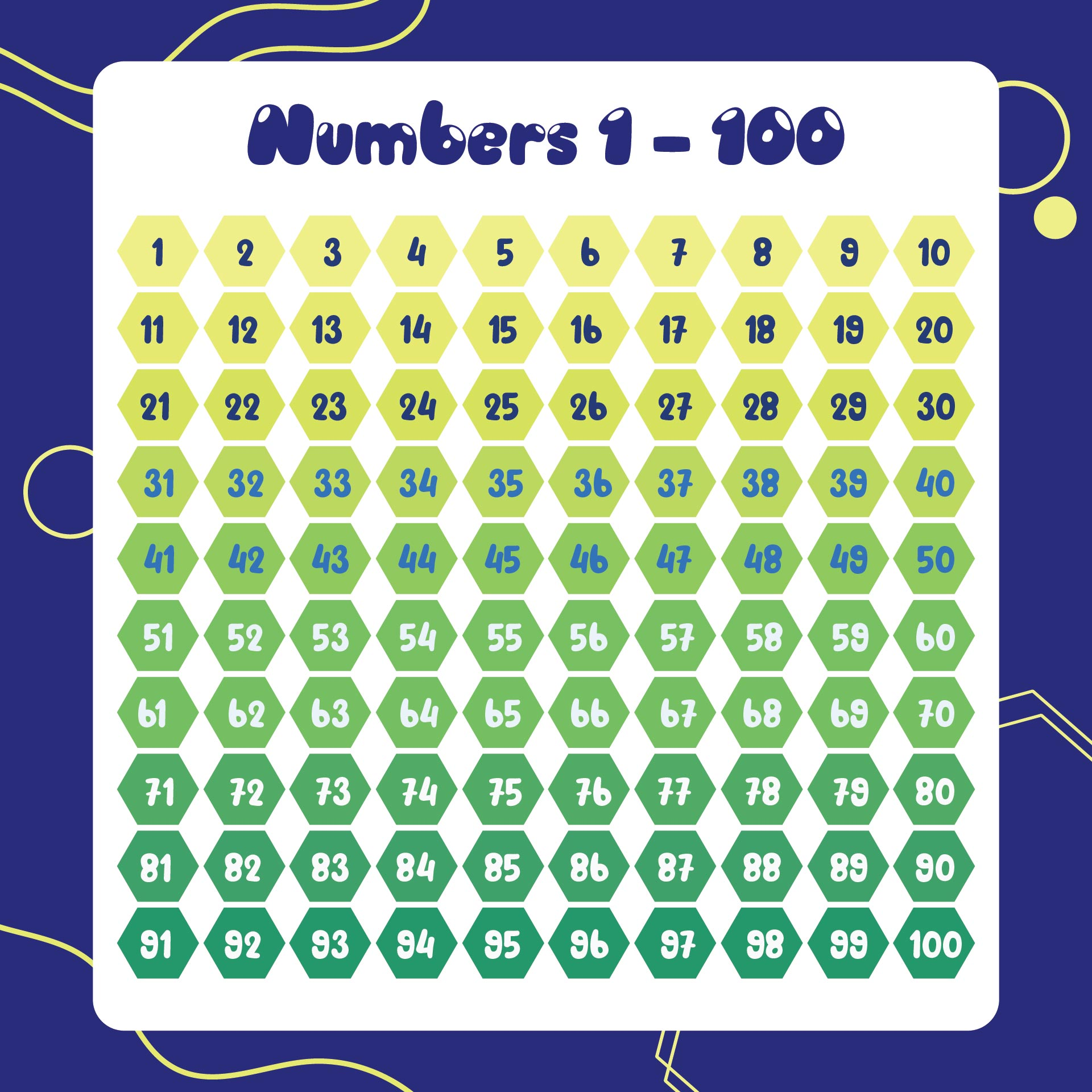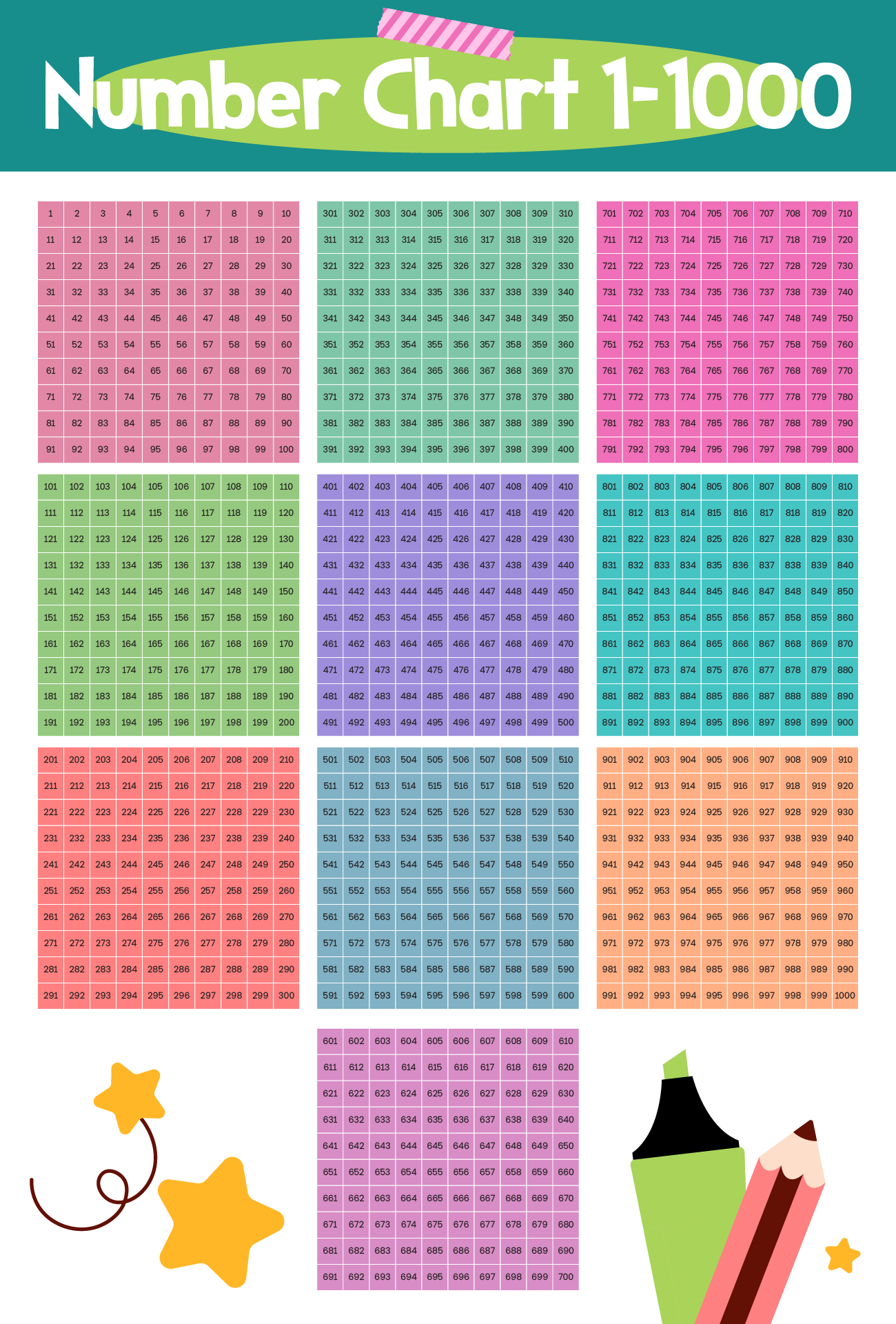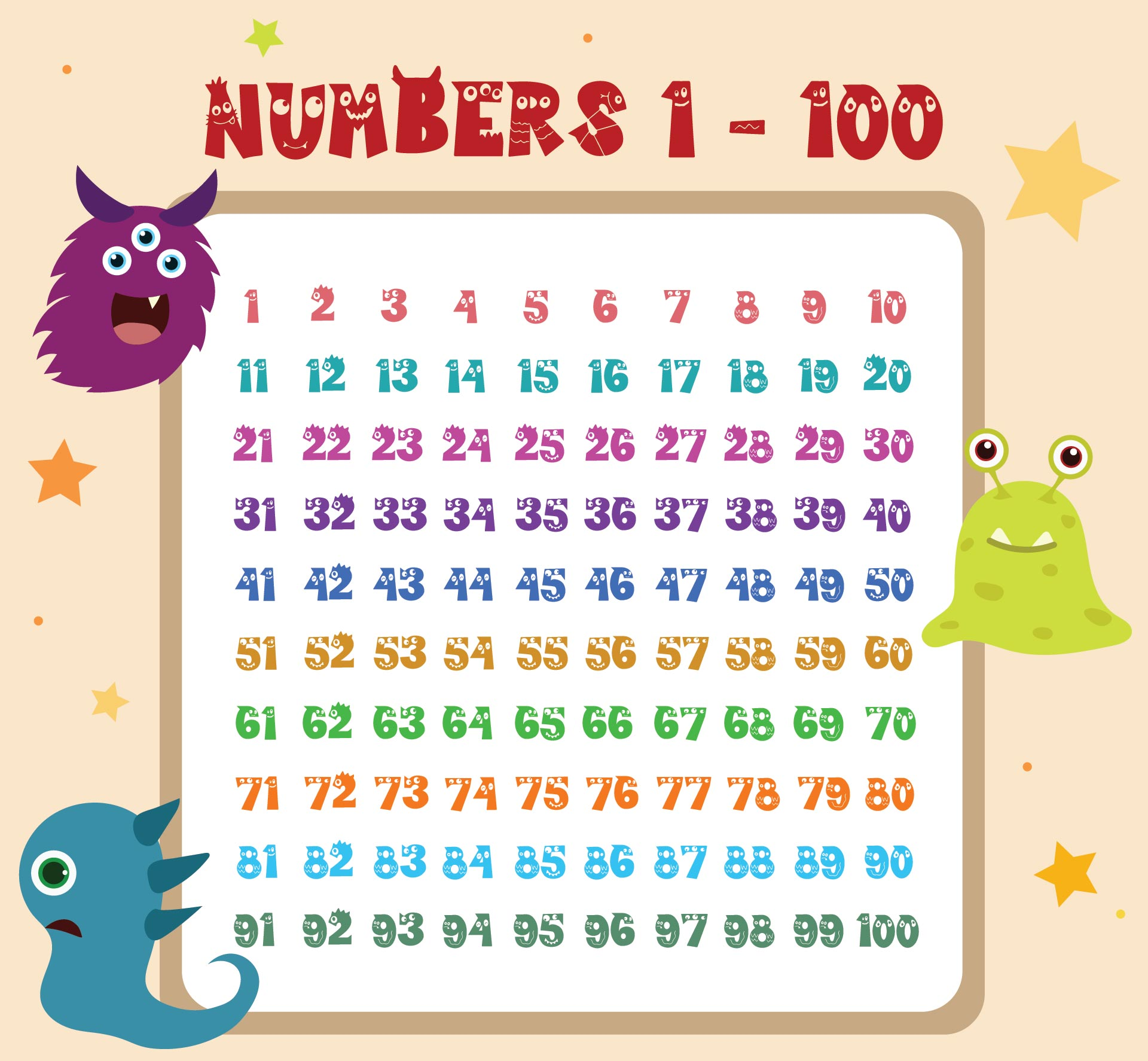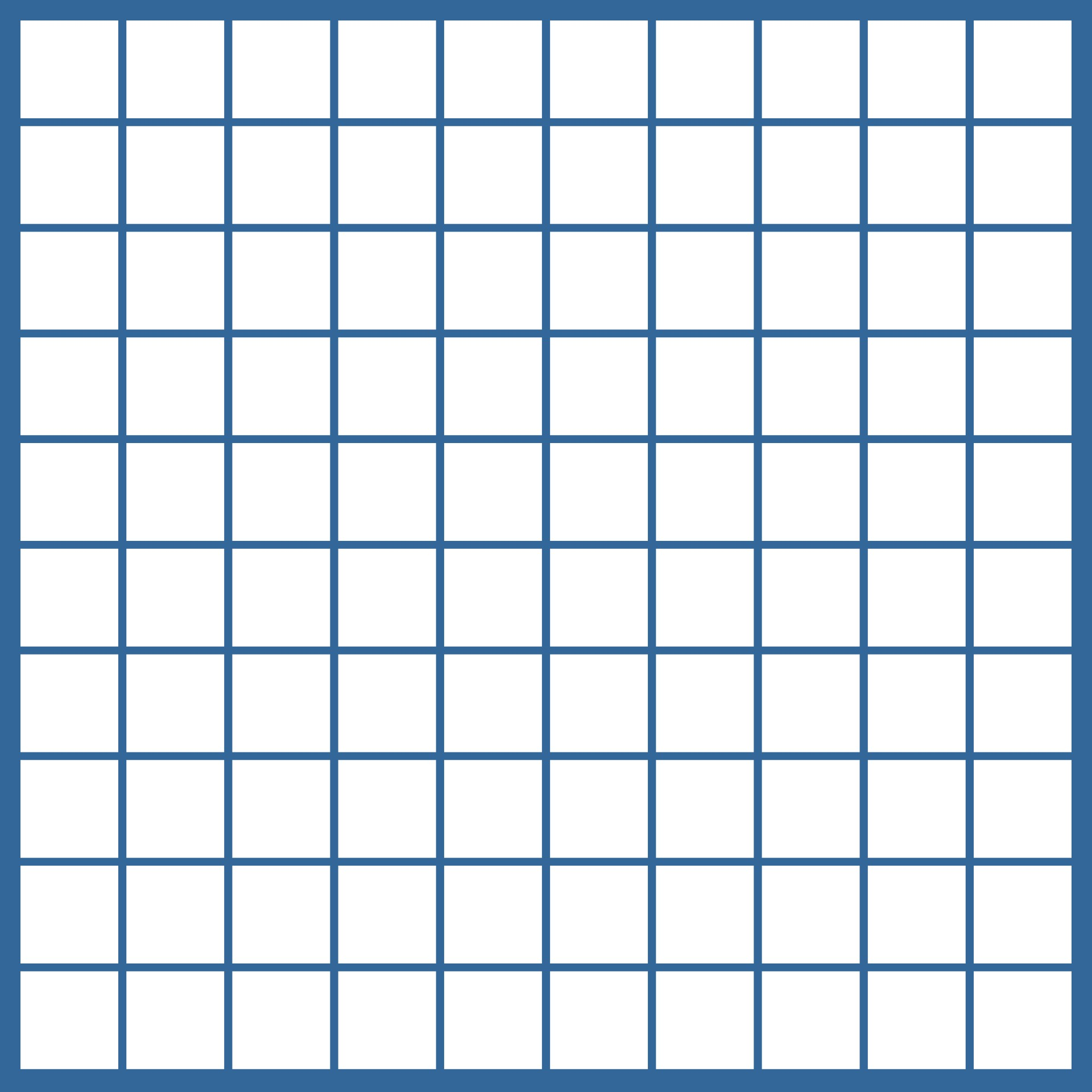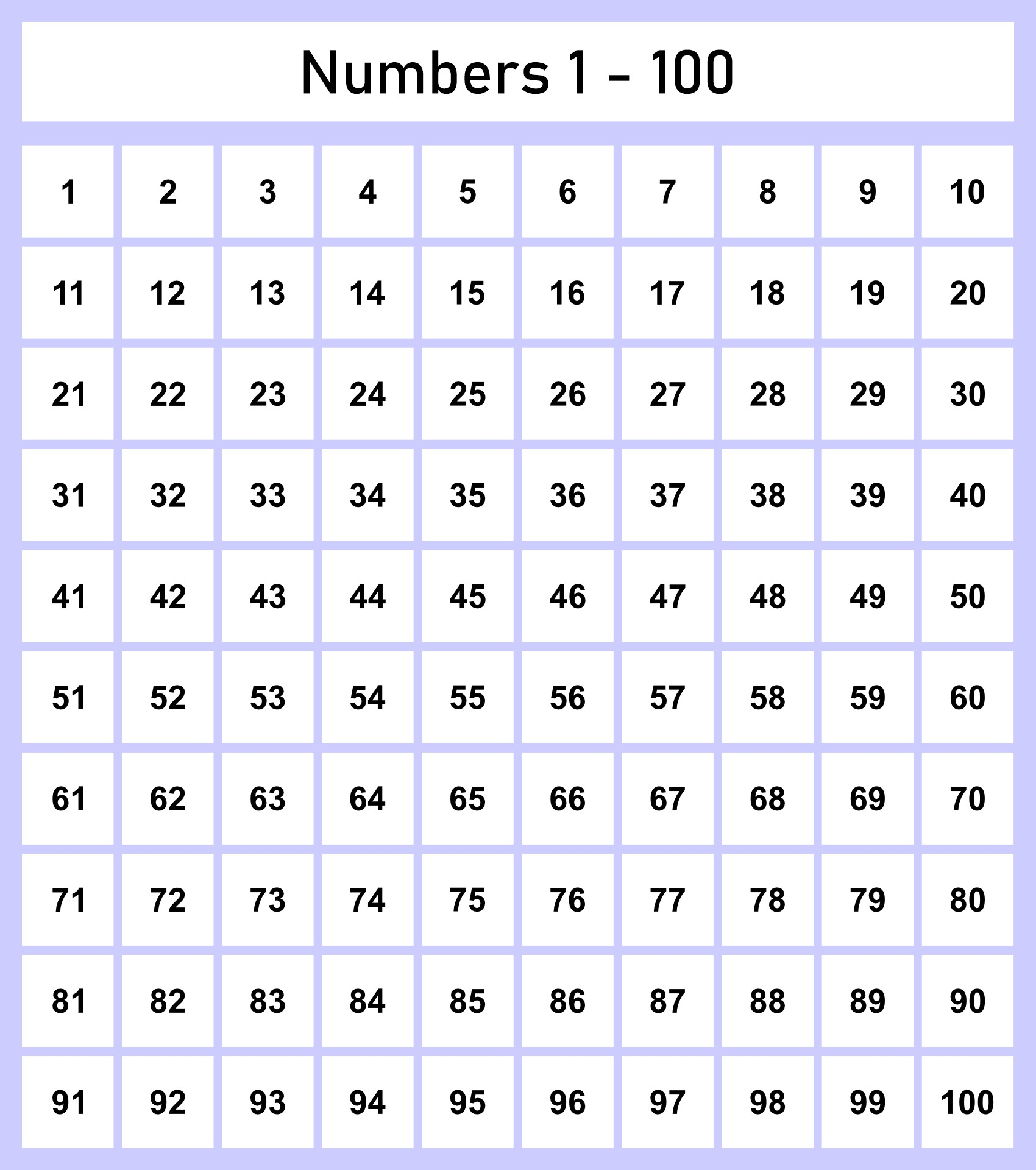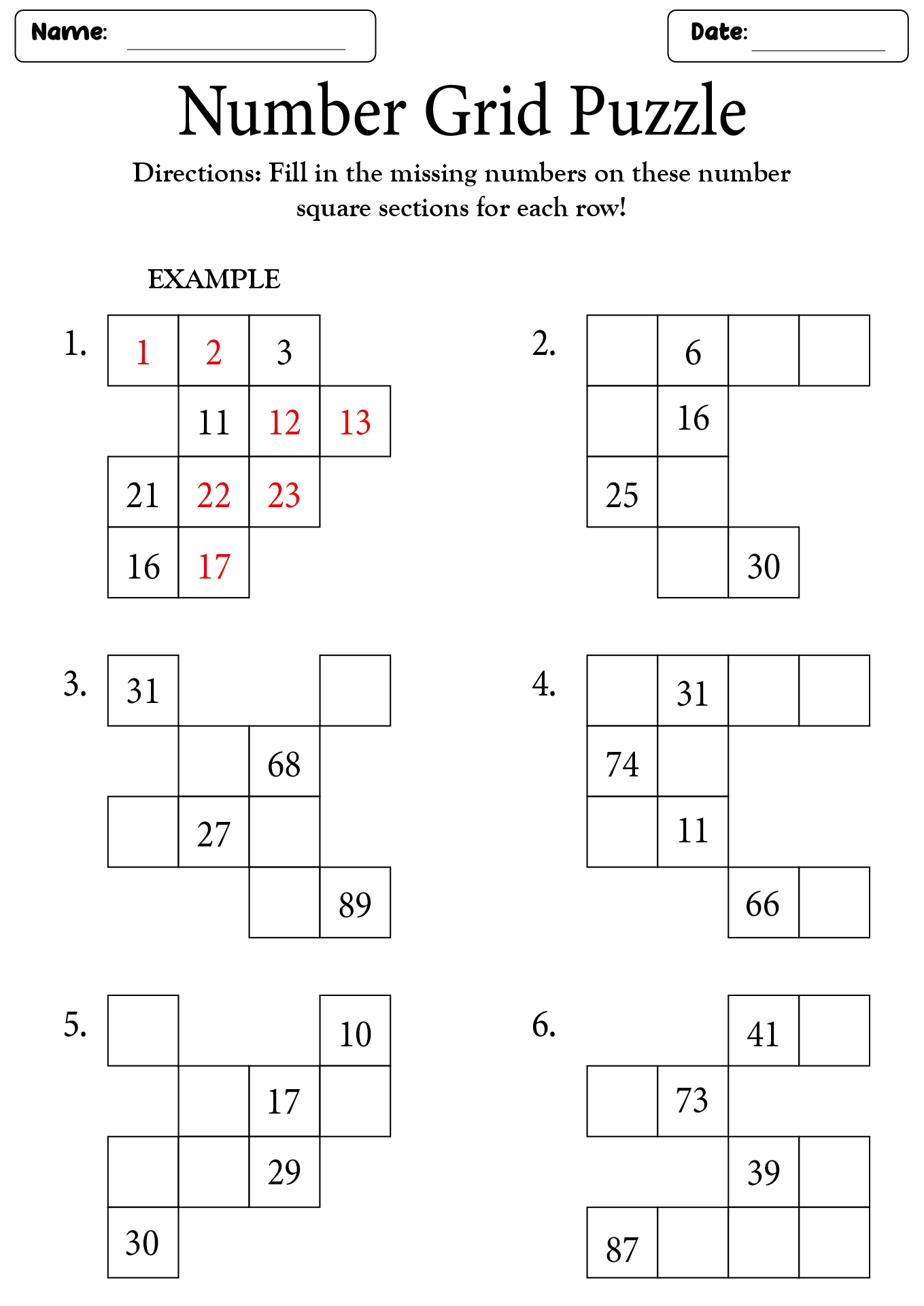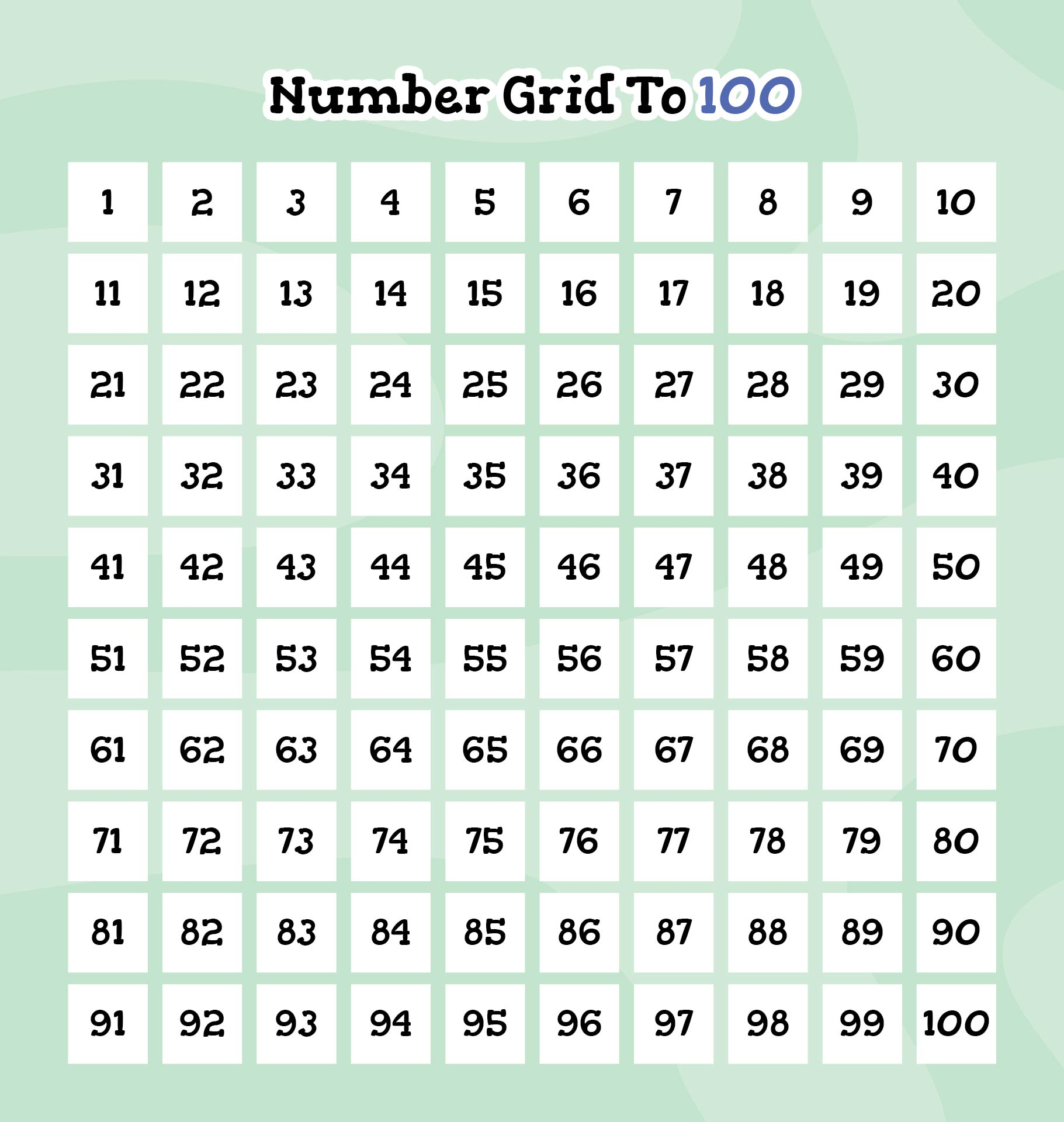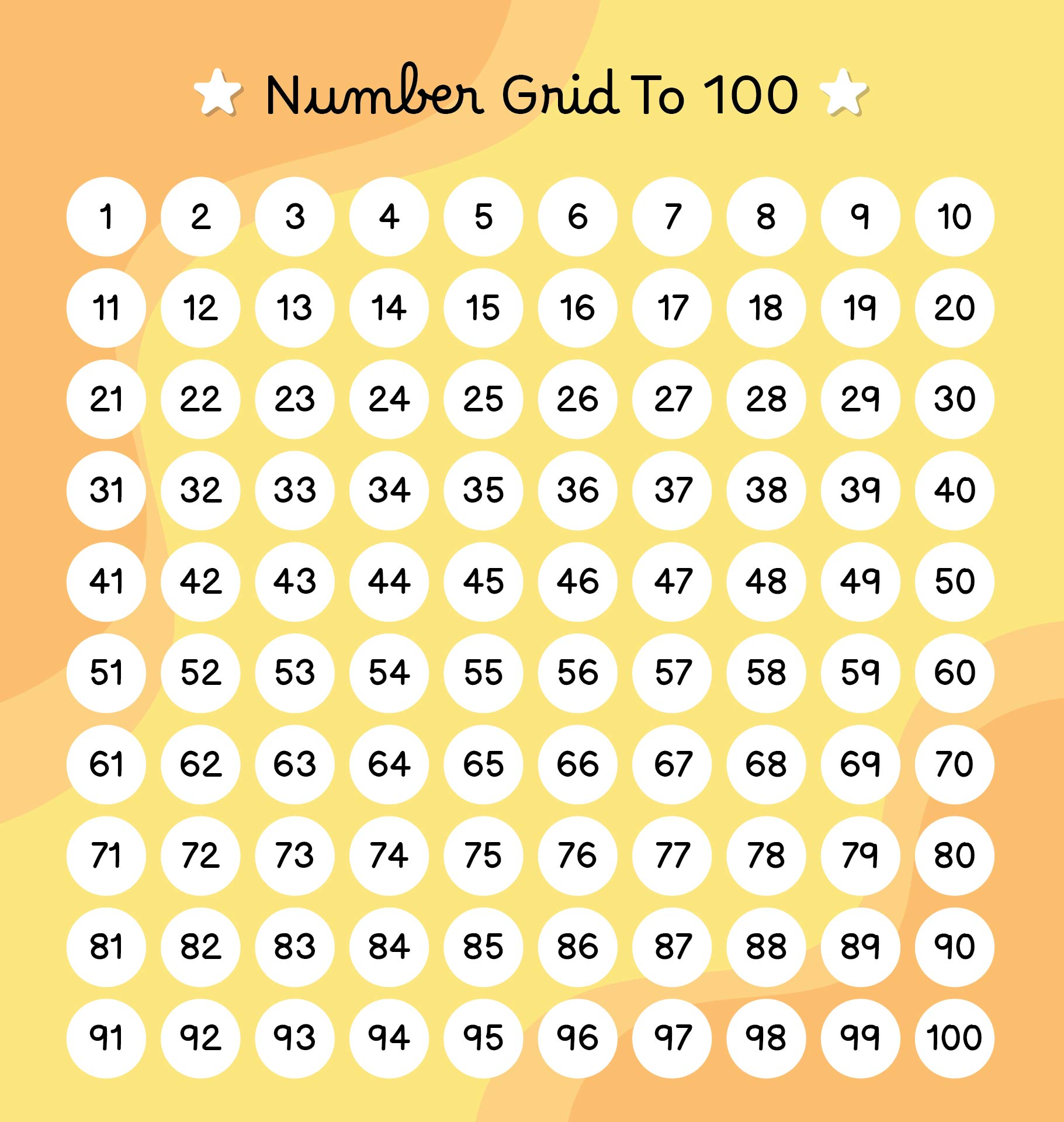## How to Use Number Grids in Upper Elementary Math?

Encourage students to interact and actively explore number grids. Give students the chance to physically manipulate the grids, color-code the numbers, or use manipulatives to draw attention to patterns through hands-on activities. This multisensory strategy improves comprehension and engagement.

By giving your student's group projects that require them to examine number grid patterns collectively, you can encourage cooperative problem-solving. Encourage strategy sharing, debates, and discussions. Communication abilities, teamwork, and the sharing of various viewpoints are all encouraged by collaborative learning.

Make applications for digital resources and interactive tools to improve the educational process. Online number grid simulations or apps can give students more chances to investigate number patterns, work out puzzles, and hone their skills in an exciting and engaging way.

Draw attention to the usefulness of number grids in everyday life. Talk about how there are patterns of numbers in nature, sports scores, and calendars. Making the connection between abstract mathematical ideas and practical applications helps students relate to and find meaning in what they are learning.

Number grids are powerful tools in upper elementary math classrooms that promote deep learning, critical thinking, and problem-solving. Educators can help students develop number sense, explore patterns, reinforce multiplication and division concepts, introduce prime and composite numbers, solve puzzles, and support data analysis by utilizing the potential of number grids.

Educators can create dynamic and engaging lessons that foster mathematical fluency and empower students to become confident problem solvers by incorporating number grids into their teaching practices.